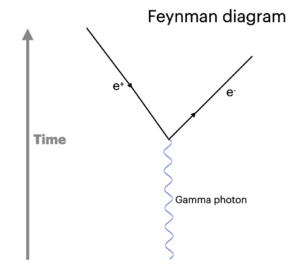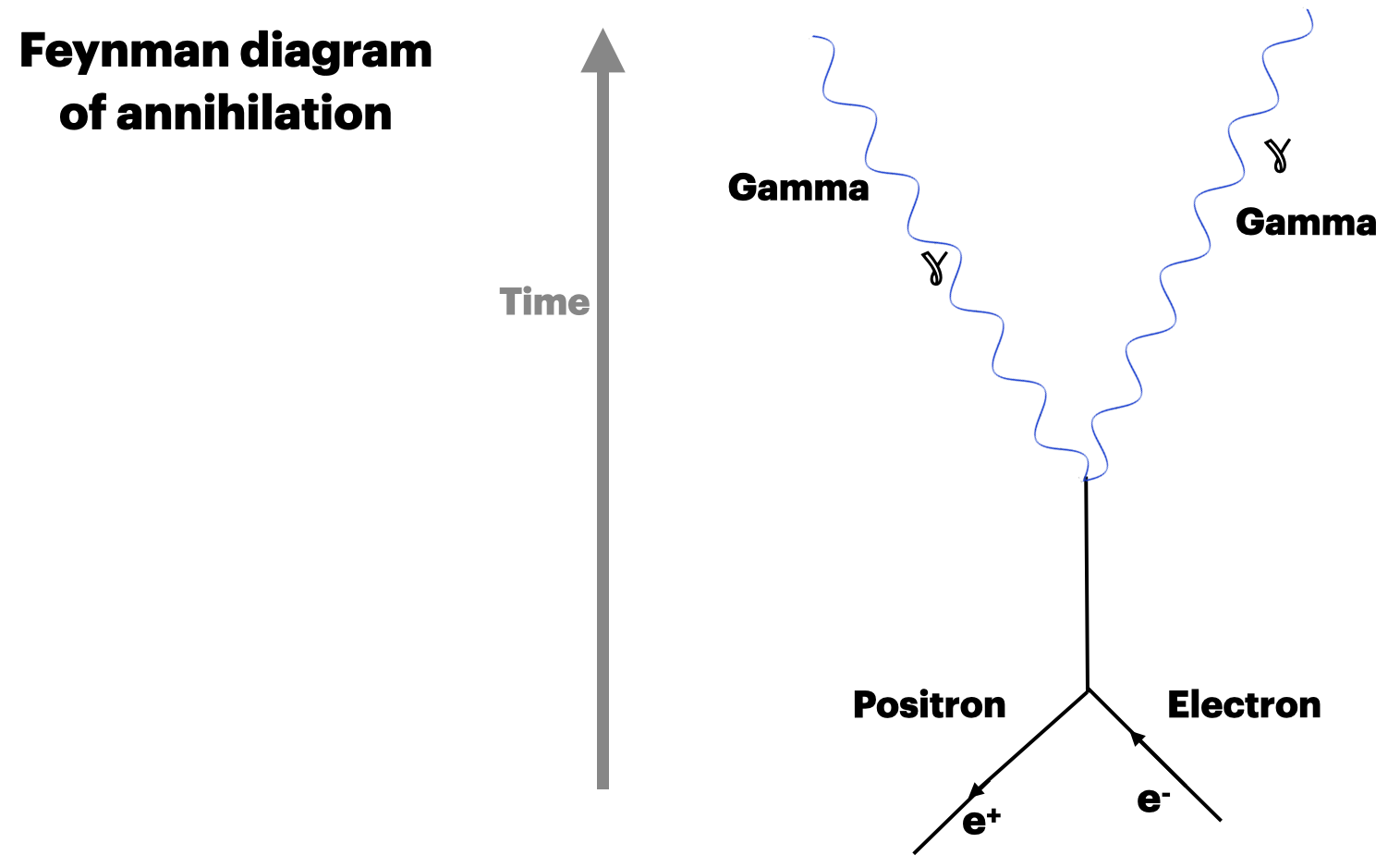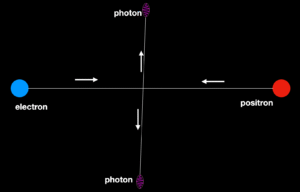### Pair production and annihilation, notes and video tutorial

In particle physics pair production and annihilation are opposite processes converting energy to particles and the reverse, all revolving around Einstein’s famous equation E=mc2

##### Pair productionA Feynman diagram of an electron and a positron being created when a high energy gamma photon interacts with an atom nucleus. Note that the arrow on the positron points towards the junction denoting that it is antimatter.

In pair production a high energy photon interacts with the nucleus of an atom producing two particles. The two have to be one of each of matter and antimatter, following the rules of conservation of lepton number, momentum, energy and strangeness.

The most common pair production is of electron and positron. More massive particles can be produced but they would require exceptionally energetic/high frequency photons.Feynman diagram of an electron and a positron annihilating each other causing the production of two photons. Note that the arrow on the positron points away from the point of collision to denote that it is antimatter.

##### Annihilation

Annihilation occurs when a particle and an anti-particle meet producing two photons. (It is always two photonsIllustration of an electron and a positron meeting and annihilating each other to produces a gamma photon

since the production of only one would break the law of conservation of momentum.)

To find out what kind of photon is needed for pair production or which photons are produced in an annihilation we have to do a couple of  calculations using, you guessed, E=mc2

##### Energy to mass

To calculate the minimum energy required to produce the mass of an electron and a positron from E=mc2

If an electron and a positron are produced then they both have a mass of 9.109

× 10-31 kilograms so that is a total of 1.822 x 10-30 kg

The minimum energy required (this does not take into account the kinetic energy of the particles produced) to do that is 1.822 x 10-30 x 9×1016

=1.63×10-13  joules

##### What kind of radiation could cause this mass to be created?

The energy of a photon is from E = hf where h is Planck’s Constant

so 1.63×10-13  = 6.62607004 × 10-34 x f

giving f = 2.46 x 1020 Hz which is well into the gamma ray band of the e/m spectrum

In the reverse annihilation – the calculation would be the same except that since the energy is split between two photons the energy of each would be halved and therefore the frequency would be halved but still well within the gamma band of radiation.